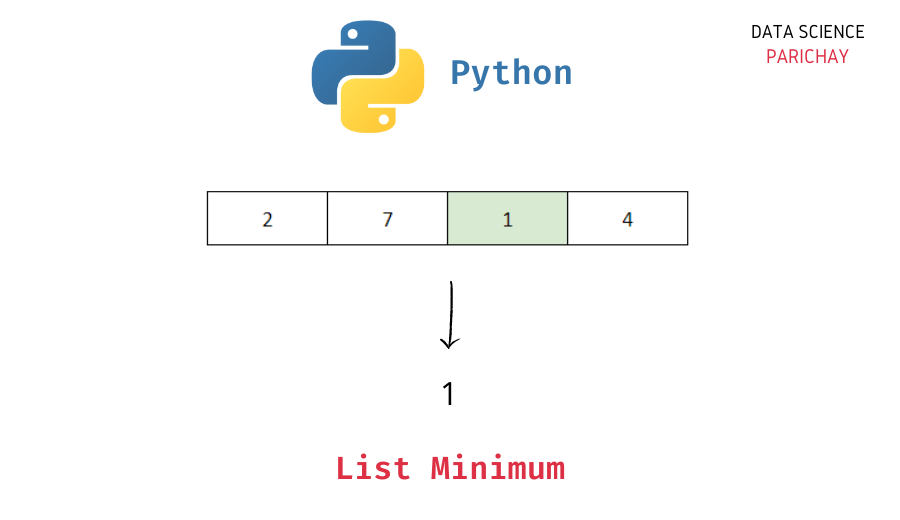# Python – Find Min Value and its index in List

In this tutorial, we will look at how to find the min value in a Python list and its corresponding index with the help of some examples.

A simple approach is to iterate through the list and keep track of the minimum value. Alternatively, you can also use the Python built-in `min()` function to find the minimum value in a list.

Let’s look at some of the different ways of finding the smallest value and its index in a list.

Iterate through the list values and keep track of the min value. Here’s an example.

```# create a list
ls = [3, 6, 7, 2, 1, 5]

# find min value using loop
min_val = ls
for val in ls:
if val < min_val:
min_val = val
# display the min value
print(min_val)```

Output:

`1`

Here, we iterate over each value in the list `ls` and keep track of the minimum value encountered in the variable `min_val`. After the loop finishes, the variable `min_val` stores the minimum value present in the list, 1.

You can use this method to get the index corresponding to the minimum value in the list as well. Use an additional variable to keep track of the current minimum value’s index.

```# create a list
ls = [3, 6, 7, 2, 1, 5]

# find min value using loop
min_val = ls
min_val_idx = 0
for i in range(len(ls)):
if ls[i] < min_val:
min_val = ls[i]
min_val_idx = i

# display the min value
print(min_val)
# display its index
print(min_val_idx)```

Output:

📚 Data Science Programs By Skill Level

Introductory

Intermediate ⭐⭐⭐

🔎 Find Data Science Programs 👨‍💻 111,889 already enrolled

Disclaimer: Data Science Parichay is reader supported. When you purchase a course through a link on this site, we may earn a small commission at no additional cost to you. Earned commissions help support this website and its team of writers.

```1
4```

We get the minimum value and its index after the loop finishes. Here we iterate through the list via its index rather than the values. You can also use the `enumerate()` function to iterate through the index and value together.

You can also use the Python built-in `min()` function to get the min value in a list. The function returns the minimum value in the passed iterable (for example, list, tuple, etc.).

```# create a list
ls = [3, 6, 7, 2, 1, 5]
# find min value
min(ls)```

Output:

`1`

Using the `min()` function is simple and is just a single line code compared to the previous example.

You can use the list `index()` function to find the index corresponding to the minimum value (assuming you already know the minimum value).

```# create a list
ls = [3, 6, 7, 2, 1, 5]

# find min value
min_val = min(ls)
# display the min value
print(min_val)
# display its index
print(ls.index(min_val))```

Output:

```1
4```

We get the min value and its index in the list `ls`.

Note that the list `index()` function returns the index of the first occurrence of the passed value. If the min value occurs more than once in the list, you’ll only get the index of its first occurrence. You can use list comprehension to get all the indices of occurrence of the min value in the list.

```# create a list
ls = [3, 6, 1, 2, 1, 5]

# find min value
min_val = min(ls)
print(min_val)
# find all indices corresponding to min val
min_val_idx = [i for i in range(len(ls)) if ls[i]==min_val]
print(min_val_idx)```

Output:

```1
[2, 4]```

We get all the indices where the minimum value occurs in the list `ls`.

You might also be interested in –

•